EnchantedLearning.com is a user-supported site.
As a bonus, site members have access to a banner-ad-free version of the site, with print-friendly pages.

 You might also like: Triangle Classification Worksheet Triangle Worksheet Triangles: Shapes Going Up at a 40-Degree Angle Triangle Shape Book: Cover Page Today's featured page: Brazil

 More Math Triangles More Shapes

A triangle is a three-sided figure. The sum of the interior angles of a triangle is 180 degrees. Each of the three points on the tips of a triangle is called a vertex (the plural of vertex is vertices).

The perimeter of a triangle is the sum of the lengths of the three sides. The area of a triangle is (1/2)*base*height.

Triangles can be classified by the length of their side or by their interior angles.

Classifying triangles by side length: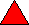equilateral triangleThe sides of an equilateral triangle are all the same length and all the interior angles are 60 degrees.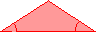isosceles triangleAn isosceles triangle has two sides that are the same length and two interior angles that are the same.scalene triangleThe sides of a scalene triangle are all different lengths. A right triangle can be a scalene triangle (but not all right triangles are scalene triangles).

The interior angles of a all triangles add up to 180 degrees. Every triangle has at least two acute angles (an acute angle is less than 90 degrees).

Classifying triangles by interior angles:acute triangleAll three interior angles of an acute triangle are acute (less than 90 degrees). Equilateral triangles are acute triangles.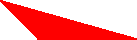obtuse triangleOne of the interior angles of an obtuse triangle is obtuse ( an obtuse angle is over 90 degrees).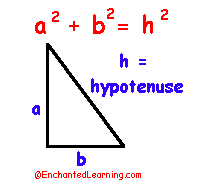right triangleA right triangle has one angle that is a right angle (a right angle is a 90-degree angle). The Pythagorean theorem states that for a right triangle, the sum of the squares of the two shorter sides is equal to the square of the hypotenuse (h): a2 + b2 = h2. The hypotenuse is the longest side of a right triangle, and is the side opposite the right angle.

Triangle Worksheets:Triangle WorksheetRead about triangles, do dot-to-dots, draw them, color them, and write the names of things with a triangular shape. Or go to the answers.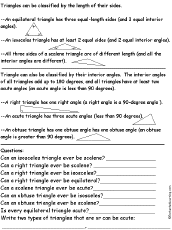Triangle Classification WorksheetRead about how to classify triangles with respect to side length and interior angles (equilateral, isosceles, scalene, right, acute, and obtuse triangles). Or go to the answers.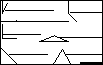Angles and TrianglesLabel the angles (acute, right, obtuse, and straight) and triangles (equilateral, isosceles, scalene, and right).Answers

Finish the Drawing Worksheets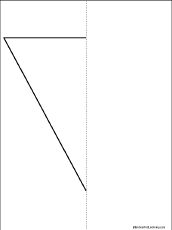Symmetrical Triangle Picture #1: Finish the Drawing Worksheet

Finish the drawing of the triangle around the line of symmetry. Or go to the answer page.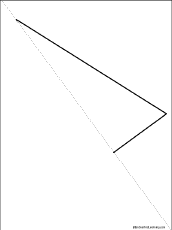Symmetrical Triangle Picture #2: Finish the Drawing Worksheet

Finish the drawing of the triangle around the line of symmetry. Or go to the answer page.Symmetrical Triangle Picture #1: Finish the Drawing and Fill in the Missing Letters Worksheet

Finish the drawing of the triangle around the line of symmetry. Or go to the answer page.Symmetrical Triangle Picture #2: Finish the Drawing and Fill in the Missing Letters Worksheet

Finish the drawing of the triangle around the line of symmetry. Or go to the answer page.

Books to PrintThings Shaped Like TrianglesA Printable Activity BookA small, triangular shaped book with triangular things to draw, including mountain, volcano, rocket, banner, and pyramid. A one-page printout.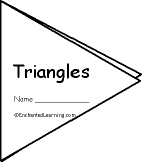TrianglesA Printable Activity BookA small, triangular shaped book about triangles, with pages: circle the triangles, draw a triangle, definition of an equilateral triangle, definition of an isosceles triangle, and definition of a right triangle. A one-page printout.

 +, - EnchantedLearning.comMath x, ÷
 A B C D E F G H I J K L M N O P Q R S T U V W X Y Z
 Number Line Fractions Decimals Measurement Rounding Graphs

Enchanted Learning®
Over 35,000 Web Pages
Sample Pages for Prospective Subscribers, or click below

 Overview of Site What's New Enchanted Learning Home Monthly Activity Calendar Books to Print Site Index K-3 Crafts K-3 Themes Little ExplorersPicture dictionary PreK/K Activities Rebus Rhymes Stories Writing Cloze Activities Essay Topics Newspaper Writing Activities Parts of Speech Fiction The Test of Time iPhone app TapQuiz Maps - free iPhone Geography Game Biology Animal Printouts Biology Label Printouts Biomes Birds Butterflies Dinosaurs Food Chain Human Anatomy Mammals Plants Rainforests Sharks Whales Physical Sciences: K-12 Astronomy The Earth Geology Hurricanes Landforms Oceans Tsunami Volcano Languages Dutch French German Italian Japanese (Romaji) Portuguese Spanish Swedish Geography/History Explorers Flags Geography Inventors US History Other Topics Art and Artists Calendars College Finder Crafts Graphic Organizers Label Me! Printouts Math Music Word Wheels

## Enchanted Learning Search

 Search the Enchanted Learning website for: